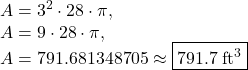Question

Find the volume (in cubic feet) of a cylindrical column with a diameter of 6 feet and a height of 28 feet. (Round your answer to one decimal place.)

1.Giakhanh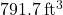The volume of a cylinder with radius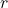and height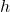is given by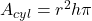.
By definition, all radii of a circle are exactly half of all diameters of the circle. Therefore, if the diameter of the circular base of the cylinder is 6 feet, the radius of it must be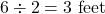.
Now we can substitute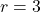and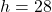into our formula: